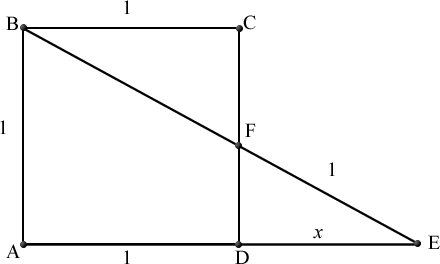# Geometric Nightmare

Geometry Level 3Diagram above shows a unit square $ABCD$, the line $AD$ is extended to point $E$ such that $BE$ is a straight line intersecting the line extended line of $AD$ at $E$ with $F$ as a point of $BF$ that is a point on line side $CD$ such that $FE = 1$. Let distance $DE$ be denoted as $x$. Find the value of $x$.

×